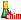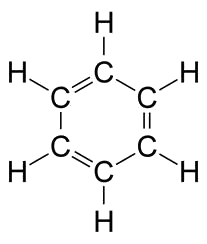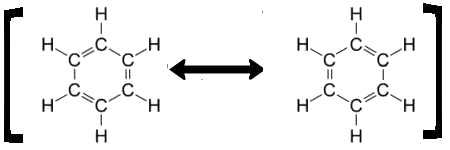Search:

# The mesomerism (resonance) of benzene

## Kekule's formula

By the mid-19th century, the chemist Kekule proposed the following structure for benzene $C_6H_6$ :## Objections

1) Substituting two $H$ atoms in benzene by chlorine atoms , we always find only three dichlorobenzenes, while we should have found four2) A single $C-C$ bond has a length of $154 \cdot 10^{-12}\; m$ , that of a double $C = C$ bond is $134 \cdot 10^{-12}\;m$. However, in benzene all the bonds have the same length: $139 \cdot 10^{-12} \; m$, intermediate between the previous two! 3) By adding one mole of $H_2$ to one mole of benzene, it wins $120\;kJ$ of energy. To hydrogenate three moles of $C = C$, it would therefore earn $360\;kJ$. However by transforming one mole of real benzene to cyclohexane, one actually wins $208\; kJ$ !## The actual structure of benzene

Admittedly benzene has neither double nor single bonds, but the bonds are "intermediate":This explains 1) that the structures 1 and 2 are the same in both cases since then the chlorine atoms are carried by carbons bound identically. 2) all bonds have the same length 3) that the energy received by complete hydrogenation of benzene would be different from what one would expect.

## The representation of the actual structure of benzeneThe actual benzene has neither the structure A , nor structure B , but an intermediate structure difficult to be represented by the traditional Lewis formulas (2 electrons = one dash!). To keep the practical Lewis representation we simply represent benzene by both Lewis structures :The image represents one molecule intermediate between the two "contributory" structures which do not represent real molecules. The double arrow does not represent an equilibrium between two (real) molecules, but indicates that the one real molecule represented is only an intermediate between these two contributory structures!

## Comparison

A child who loves fairy tales never saw a rhino. You want to explain him what a rhino looks like. So you say that a rhinoceros (which exists really) is somehow an intermediary between a unicorn and a dragon (which the child knows, but which do not exist):## Vocabulary

Mesomerism or resonance is known from a chemical species (molecule, ion or radical) that is an intermediary between several imaginary contributory forms .